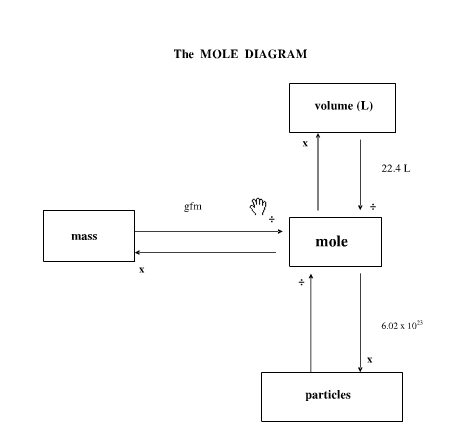##### Quantitative Chemistry

0
Set Details Share
created 10 years ago by 1farina
79 views
Formulas and Definitions
updated 10 years ago by 1farina
Subjects:
chemistry
Page to share:
Embed this setcancel
COPY
code changes based on your size selection
Size:
X
1

THE IDEAL GAS EQUATION

pV = nRT

2

'p' in the Idel Gas Equation

pressure, Pa/Nm^-2

3

'V' in the Ideal gas Equation

volume, m^3

4

'n' in the Ideal Gas Equation

number of moles

5

'R' in the Ideal Gas Equation

gas constant - 8.314 J K^-1 mol^-1

6

'T' in the Ideal Gas Equation

temperature, K

7

Boyle's Law

Pressure increases when volume decreases (temperature is kept constant)

Pressure and volume of gas are inversely proportional

8

Charle's Law

Volume increases when temperature increases (pressure is kept constant)

Volume and temperature of gas are directly proportional

9

Pressure Law

Pressure increases when temperature increases (volume is kept constant)

Temperature is directly proportional to pressure

10

Pressure Conversion

atm to Pa ?

multiply by 101 325 Pa

11

Pressure Conversion

bar to Pa ?

multiply by 10^5 Pa

12

Temperature Conversion

from degree celsius to Kelvin ?

degree celsius + 273

13

Temperature Conversion

from Kelvin to degree celsius ?

K - 273

14

Volume Conversion

from dm^3 to m^3 ?

divide by 1000

15

Volume Conversion

from cm^3 to m^3 ?

divide by 1000000 m^3

16

Concentration of solution depends on

amount of solute and volume of solvent

17

The Mole Diagram18

Mass of a single atom of an element

molar mass divided by Avogadro's Constant

19

1 mole of something contains..

6.02 x 10^23 molecules